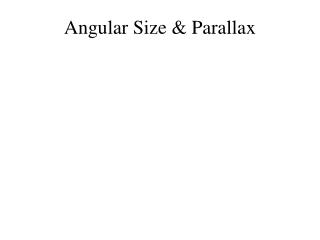DownloadDownload PresentationAngular Size & Parallax

# Angular Size & Parallax

Download Presentation## Angular Size & Parallax

- - - - - - - - - - - - - - - - - - - - - - - - - - - E N D - - - - - - - - - - - - - - - - - - - - - - - - - - -
##### Presentation Transcript

1. Angular Size & Parallax

2. Fig. 1

3. Fig. 4 10 cm wide 20 cm 10 cm wide X

4. 5 cm 5 cm 10 cm wide 4 cm high 8 cm total 4 cm high Fold on dashed lines Cut along solid black line to make a strip. Fold on dashed. Cut object template and “Paper Mate Sharpwriter” holder from part of an 8 ½ x 11 inch sheet of paper as shown here. Fig. 5 Object template

5. Fig. 5 lower. Assembled ang. Size object and parallax target • Tape pencil to middle of 10 cm wide object. • Make object which holds pencil 4 cm high. • Dashed line divides triangle symmetrically. 10 cm wide To observer

6. Example: d=115 cm angular size= 6o. Phy.size th.=115cm(6o/57.3o) = 12 cm Actual size =13 cm =100(13-12)/13=8%

7. Aristarchus example • Thought Sun distance 840x diameter Earth • About 0.5o angular size • Physical size =840(0.5/57.3)= 7xdiameter Earth. => Earth orbits Sun, Was ignored. Modern distance ≈ 12000 diameter Determined by parallax

8. Aristarchus’ ideas were rejected. Why? • Common sense AND failure to observe stars’ parallax.

9. Parallax used to get distances of solar system objects and stars. • Fig. 6 Geometry of parallax experiment set-up

10. Layout for parallax observation versus theory Two baseline points are 10 cm on each side of line of "x's". 10 cm wide X 20 cm

11. Layout for parallax observation versus theory Two baseline points are 10 cm on each side of line of "x's". 10 cm wide

12. Viewing parallax target with pinhole protractor • Nearby pencil relative to distant star along dashed line. • From each of two baseline points 10 cm from “Sun.” Pencil target to distant star.

13. Figure 9-Protractor views of nearby star (red) vs distant star (blue). • View of angular scale as seen through protractor. • Lower example: p = (50-24)/2= 13o. • Upper: p= (25+25)/2= 25o.

14. Lower example previous page: p= 13o. R= 10 cm • d = 57.3 o (10/13 o) = 44.1 cm • Actual d = 43.3 cm • % error=100(44.1-43.1)/43.3 ≈ 2%

15. Finding the Distance of our Sun) • Recall Copernicus found relative distances of planets in solar system wrt Earth-Sun 1 AU. • Measure parallax of Earth-Sun, Earth-Planets etc • Observe from one side of Earth to other • 93 million miles=150 million km.

16. The Long Quest for Stellar Parallax • Not until 1800’s. • German astronomer Karl Bessel • First accurate measurement of parallax of star 61 Cygni in 1838. • Tiny, p = 0.3 seconds of arc. One arc sec= 1/3600 o

17. Distance pc=1/p parallax in arc sec1 arc sec = 1/3600 degree1 pc=200,000 AU=3.26 LY. 1 LY = 6trillion miles

18. Even the nearest stars are amazingly far away. • Nearest star parallax, • p ~0.7725 arc sec • 1/0.7725=1.3 pc away, about four light years travel time. • > 200,000 times Earth-Sun distance • The double star, Alpha & Proxima Centaurus.

19. Example calculation of distance with parallax. • A star’s Π = 0.5 sec of arc. • Distance in parsec = 1/Π in arc sec. • Distance = 1/0.5 = 2 parsecs = 6.52 light years or about 400,000 AU = 39 trillion miles.

20. Progress in Measuring Parallax • Limited by atmosphere “seeing.” and instrument. • Bessel could measure distances to ~8 pc, ~25 ly, only a few neighboring stars. • 1989 Hipparchus satellite, to 0.001 arc sec; d=1000pc= 3000 ly. • Still only 3% of our Galaxy. • 2011 Gaia space probe, will cover our whole Galaxy.

21. End of Presentation